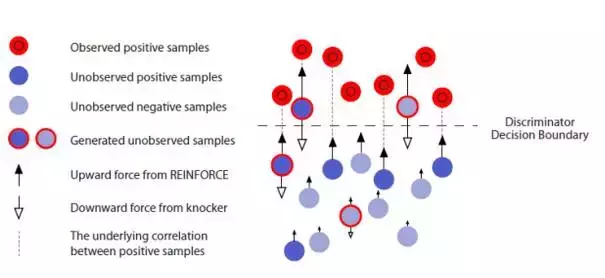Auto ByteScience AI# 引入秘密武器强化学习，发掘GAN在NLP领域的潜力（附公开课）

1、基础：文本生成模型的标准框架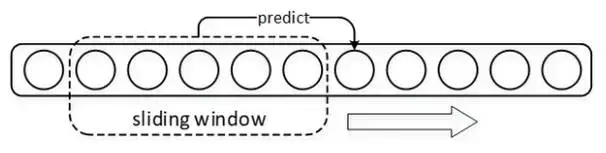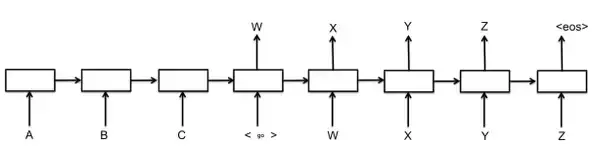2、问题：GAN为何不能直接用于文本生成

2.1GAN基础知识

GAN对于大家而言想必已经脍炙人口了，这里做一些简单的复习。

GAN从结构上来讲巧妙而简单（尽管有与其他经典工作Idea相似的争议[6~7]），也非常易于理解，整个模型只有两个部件：1.生成器G；2.判别器D。

GAN模型的大体框架如下图所示：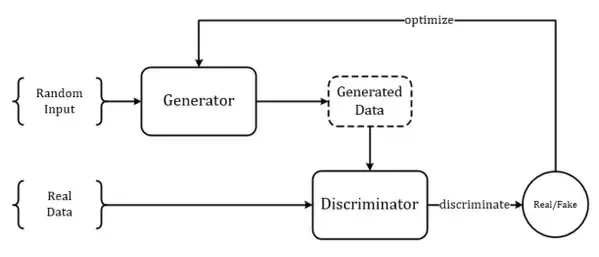\arg\min_{G}\max_{D}=V(G,D)

V=\mathbb{E}_{x \sim p_{data}}[\log D(x)] + \mathbb{E}_{x \sim p_g}[\log (1-D(x))]

V=\int_{x}\left[ p_{data}(x) \log D(x) + p_g(x) log(1-D(x))\right]

D^*(x)=\frac{p_{data}(x)}{p_{data}(x) + p_g(x)}

\begin{aligned} V(G,D^*) &= \int_x p_{data}(x)\log \frac{p_{data}(x)}{p_{data}(x) + p_g(x)} + \int_x p_g \log \frac{p_g(x)}{p_{data}(x) + p_g(x)}\\ &= -\log 4+ KL\left( p_{data}(x) \Arrowvert \frac{p_{data}(x) + p_g(x)}{2} \right) + KL\left( p_g(x) \Arrowvert \frac{p_{data}(x) + p_g(x)}{2} \right)\\ &= -\log 4 + 2\cdot JSD(p_{data}(x) \Arrowvert p_g(x)) \end{aligned}

2.2GAN面对离散型数据时的困境（啥是离散型数据？）

GAN的作者早在原版论文时就提及，GAN只适用于连续型数据的生成，对于离散型数据效果不佳（使得一时风头无两的GAN在NLP领域一直无法超越生成模型的另一大佬VAE）。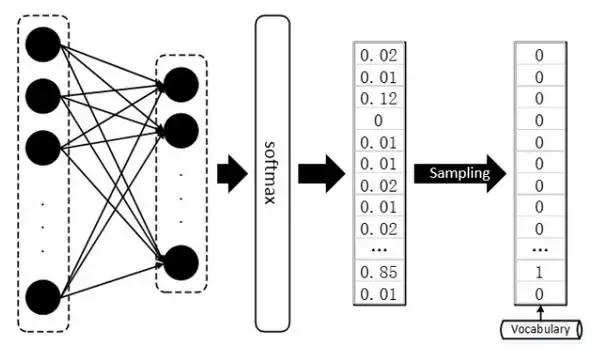JSD(P \Arrowvert Q) \equiv \log{2}

3、过渡方案：对于GAN的直接改进用于文本生成

3.1Wasserstein-divergence，额外的礼物

Wasserstein GAN（简称WGAN），其影响力似乎达到了原版GAN的高度，在国内也有一篇与其影响力相当的博文——“令人拍案叫绝的Wasserstein GAN”，不过在看这篇论文之前，还要推荐另外一篇论文“f-GAN”，这篇论文利用芬切尔共轭（Fenchel Conjugate）的性质证明了任何 f-Divergence 都可以作为原先GAN中 KL-Divergence （或者说 JS-Divergence ）的替代方案。 f-GAN 的定义如下：

D_f(P \Arrowvert Q) = \int_{x} q(x) f\left( \frac{p(x)}{q(x)} \right) dx

\left\{ \begin{aligned} & f \ is \ convex \quad (凸函数) \\ & f(1) = 0 \end{aligned} \right.

Wasserstein GAN 对GAN的改进也是从替换 KL-Divergence 这个角度对GAN进行改进，其详细的妙处大可参看文献[10,13]，总的来说，WGAN采用了一种奇特的 Divergence—— “推土机-Divergence”， Wasserstein-Divergence 将两个分布看作两堆土，Divergence 计算的就是为了将两个土堆推成一样的形状所需要泥土搬运总距离。如下图：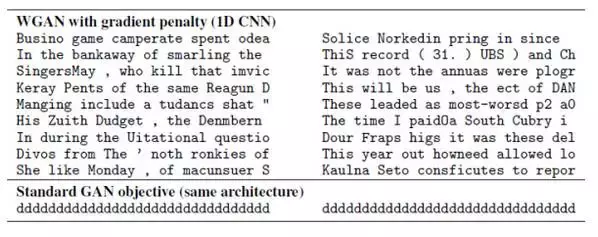3.2Gumbel-softmax，模拟Sampling的softmax

\mathrm{y} = one\_hot\left( \arg\max \left( softmax(\mathrm{h}) \right) \right)

\mathrm{y} = softmax \left( 1 / \tau (\mathrm{h} + \mathrm{g}) \right)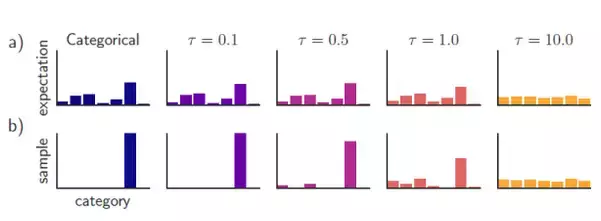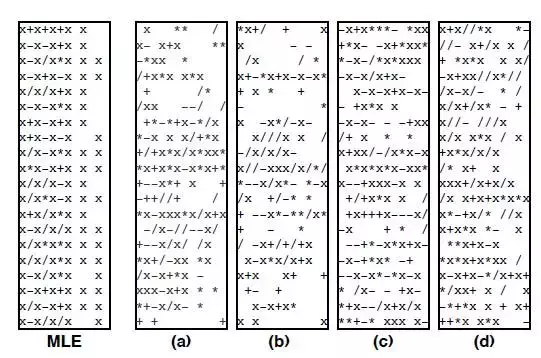4、RL在GAN文本生成中所扮演的作用

4.1关于Reinforcement Learning的闲聊闲扯

RL通常是一个马尔科夫决策过程，在各个状态 s_i 下执行某个动作 x_i 都将获得奖励（或者是"负奖励"——惩罚） Reward(s_i, x_i) ，而将从头到尾所有的动作连在一起就称为一个“策略”或“策略路径” \theta^\pi ，强化学习的目标就是找出能够获得最多奖励的最优策略：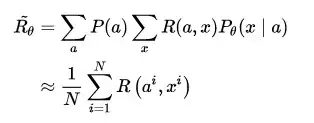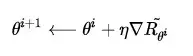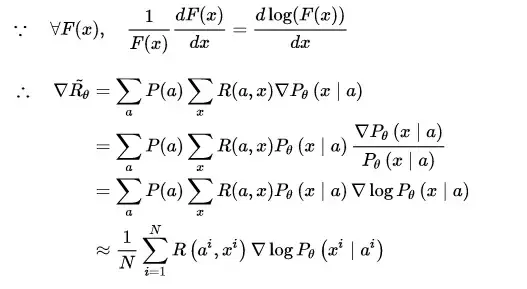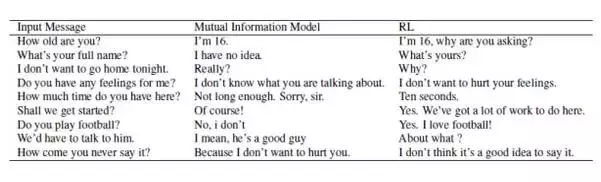4.2SeqGAN 和 Conditional SeqGAN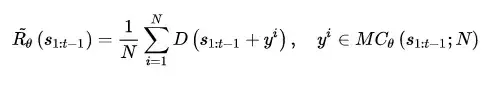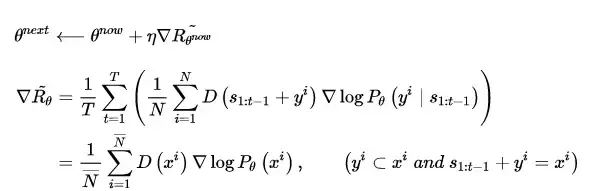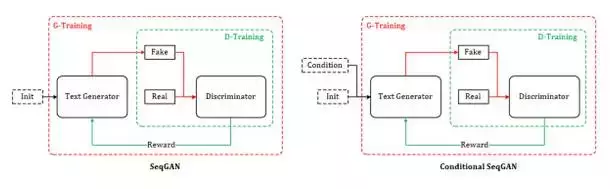SeqGAN的提出为GAN用于对话生成（Chatbot）完成了重要的铺垫，同样起到铺垫作用的还有另外一个GAN在图像生成领域的神奇应用——Conditional GAN[18~19]，有条件的GAN，顾名思义就是根据一定的条件生成一定的东西，该工作根据输入的文字描述作为条件，生成对应的图像，比如：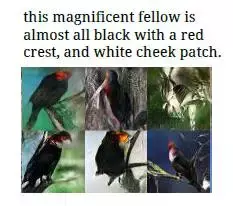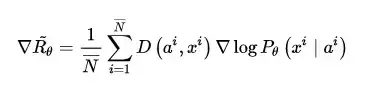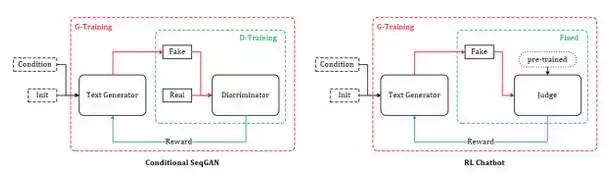RL+ GAN 利用强化学习中的 Reward 机制以及 Policy Gradient 等技术，巧妙地避开了GAN面对离散数据时梯度无法BP的难题，在使用强化学习的方法训练生成器G的间隙，又采用对抗学习的原版方法训练判别器D。 在Conditional SeqGAN对话模型的一些精选结果中，RL+ GAN 训练得到的生成器时常能返回一些类似真人的逼真回答（我真有那么一丝丝接近“恐怖谷”的感受）。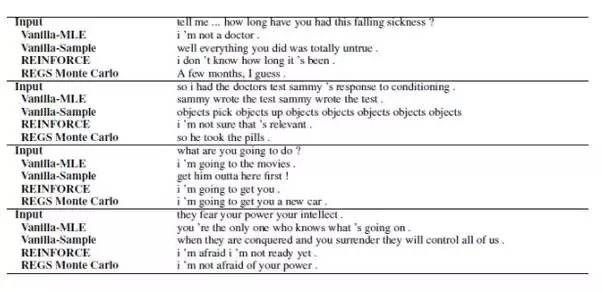5、一些细节 + 一些延伸

5.1Reward Baseline：奖励值上的 Bias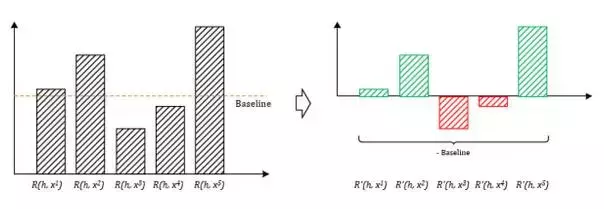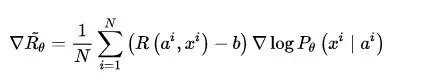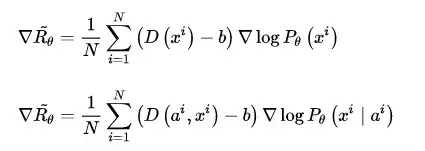5.2REGS：一人犯错一人当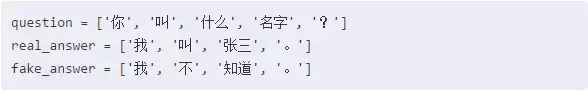P_{\theta}\left(x^i \mid a^i \right) = P_{\theta}\left( x^i \bracevert a^i \right) + P_{\theta}\left( x^i \bracevert a^i, x^i \right) + \dots + P_{\theta}\left( x^i[T] \bracevert a^i, x^i[1:T-1] \right)

\nabla\tilde{R_{\theta}} = \frac{1}{N} \sum\limits_{i=1}^{N} \sum\limits_{t=1}^{T} \left(D_e\left(a^i, x^i[1:t] \right) - b\right) \nabla\log P_{\theta}\left(x^i[t] \mid a^i, x^i[1:t-1] \right)

5.3MC Search & Discriminator for Partially Decoded Sequences：准度与速度的抉择

D_e\left(a^i, x^i[1:t] \right) = \frac{1}{M} \sum\limits_{p=1}^{M}\left(D\left(a^i, x^i[1:t] + x_r^p[t+1:T] \right) \right)

5.4Teacher Forcing：给Generator一个榜样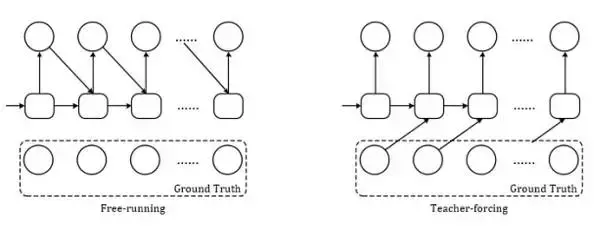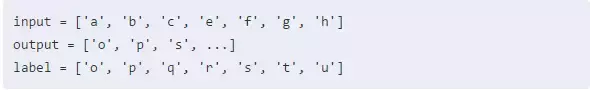Free-running mode下的decoder会将第三项错误的输出 output = 's'（下标从0开始）作为下一个state的输入，而在Teacher-forcing mode下，decoder则会将正确样本的第三项 label = 'q' 作为下一个state的输入。 当然这么做也有它的缺点，因为依赖标签数据，在training的时候会有较好的效果，但是在testing的时候就不能得到ground truth的支持了。最好的结果是将Free-running mode的behavior训练得尽可能接近于Teacher-forcing mode，Professor Forcing使用GAN尝试实现了这一目标。

SeqGAN也存在这样的问题，一开始的生成器G非常弱，即便是经过一定量的预训练，也几乎生成不出好的Result，然后这些bad result给到鉴别器D必然只能返回很低的 Reward （惩罚），生成器G的训练只能根据鉴别器的打分来优化而无法得到good example的指导，永远不知道什么是好的结果，结果必然是恶性循环。于是，有必要在SeqGAN训练中给到生成器G真实样本的指导，也就是告诉生成器：“什么样的样本才配得到高分 Reward ?”

4.2节中提到，生成器G 和 判别器D的训练时交替进行的，由于鉴别器返回的打分是判定输入样本为真的概率，我们可以随机取出一部分真实的样本对话组 \mathrm{Real} = \left\{ \left(a_r^0, x_r^0 \right), \left(a_r^1, x_r^1 \right), \cdots, \left(a_r^n, x_r^n \right) \right\} ，然后直接设置他们的鉴别器奖励值为 1 （或者其他任意定义的最高分），将它们加入生成器G的训练过程中，这样生成器就能知道何种样本能得到最高的奖励，从而一定程度上避免了SeqGAN的训练过程由于一方的弱势而发生崩塌。

D\left(a_r^i, x_r^i\right) = 1, \qquad \left(\left(a_r^i, x_r^i\right) \in \mathrm{Real} \right)

D\left(a_r^i, x_r^i\right) > b, \qquad \left(\left(a_r^i, x_r^i\right) \in \mathrm{Real} \right)

5.5Actor-Critic：更广义上的GAN？

D^* = \arg\min_D -\mathbb{E}_{x \sim p_{data}}[\log D(x)] - \mathbb{E}_{z \sim \mathcal{N}\left(0, I\right)}[\log (1-D(G(z)))]

G^* = \arg\min_G \mathbb{E}_{z \sim \mathcal{N}\left(0, I\right)}[\log (1-D(G(z)))] = \arg\min_G -\mathbb{E}_{z \sim \mathcal{N}\left(0, I\right)}[\log (D(G(z)))]

Q^{\pi} = \arg\min_Q \mathbb{E}_{s_t, a_t \sim \pi}\left[\mathcal{D}\left(\mathbb{E}_{s_{t+1}, r_t, a_{t+1}} \left[r_t + \gamma Q\left(s_{t+1}, a_{t+1} \right) \right] \Arrowvert Q(s_t, a_t) \right) \right]

{\pi}^{\ast} = \arg\max_{\pi} \mathbb{E}_{s_0 \sim p_0, a_0 \sim \pi}\left[Q^{\pi}\left(s_0, a_0 \right) \right] = \arg\min_{\pi} -\mathbb{E}_{s_0 \sim p_0, a_0 \sim \pi}\left[Q^{\pi}\left(s_0, a_0 \right) \right]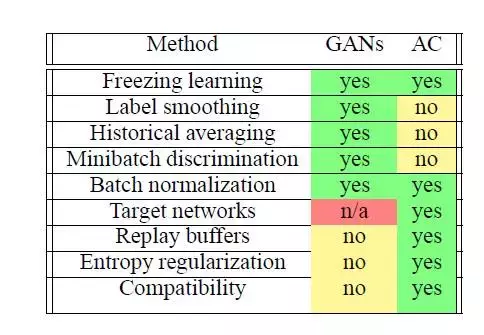5.6IRGAN：两个检索模型的对抗

IRGAN这篇工作发表于2017年的SIGIR，从作者的阵容来看就注定不是一篇平凡的作品，其中就包含SeqGAN的原班人马，作者将生成对抗网络的思想应用于信息检索领域，却又不拘泥于传统GAN的经典Framework，而是利用了IR领域原本就存在的两种不同路数的model：生成式IR模型 和 判别式IR模型。

J^{G^\ast, D^\ast} = \min_{\theta} \max_{\phi} \sum\limits_{n=1}^N \left(\mathbb{E}_{d \sim p_{real}\left(d \mid q_n,r \right)}\left[\log D\left(d \mid q_n \right) \right] + \mathbb{E}_{d \sim p_{\theta}\left(d \mid q_n,r \right)}\left[\log \left(1 - D\left(d \mid q_n \right) \right)\right] \right)

D\left(d \mid q \right) = \sigma \left(f_\phi \left(d,q \right) \right) = \frac{\exp \left(f_\phi \left(d,q \right) \right)}{1 + \exp \left(f_\phi \left(d,q \right) \right)}

\phi^\ast = \arg\max_{\phi} \sum\limits_{n=1}^N \left(\mathbb{E}_{d \sim p_{real}\left(d \mid q_n,r \right)}\left[\log \left(\sigma\left(f_\phi\left(d, q_n \right)\right)\right) \right] + \mathbb{E}_{d \sim p_{\theta}\left(d \mid q_n,r \right)}\left[\log \left(1 - \sigma\left(f_\phi\left(d, q_n \right)\right) \right)\right] \right)

\theta^\ast = \arg\min_{\theta} \sum\limits_{n=1}^{N} \mathbb{E}_{d \sim p_{\theta}\left(d \mid q_n, r \right)}\left[\log\left(1 + \exp\left(f_\phi \left(d, q_n \right) \right) \right) \right]

\begin{aligned} \nabla_\theta J^G \left(q_n \right) &= \nabla_\theta \mathbb{E}_{d \sim p_\theta \left(d \mid q_n, r \right)} \left[\log\left(1 + \exp\left(f_\phi \left(d, q_n \right) \right) \right) - b \right] \\ &= \sum\limits_{i=1}^{M} \nabla_\theta p_\theta \left(d_i \mid q_n, r \right) \left[\log\left(1 + \exp\left(f_\phi \left(d_i, q_n \right) \right) \right) - b \right] \\ &= \sum\limits_{i=1}^{M} p_\theta \left(d_i \mid q_n, r \right) \nabla_\theta \log p_\theta \left(d_i \mid q_n, r \right) \left[\log\left(1 + \exp\left(f_\phi \left(d_i, q_n \right) \right) \right) - b \right] \\ &\simeq \frac{1}{K} \sum\limits_{k=1}^{K} \nabla_\theta \log p_\theta \left(d_k \mid q_n, r \right) \left[\log\left(1 + \exp\left(f_\phi \left(d_k, q_n \right) \right) \right) - b \right] \end{aligned}

b = \mathbb{E}_{d \sim p_\theta \left(d \mid q_n, r \right)} \left[\log\left(1 + \exp\left(f_\phi \left(d, q_n \right) \right) \right) \right]

D\left(\langle d_i, d_j\rangle \mid q \right) = \sigma \left(f_\phi \left(d_i, q \right) - f_\phi \left(d_j, q \right)\right) = \frac{\exp \left(f_\phi \left(d_i, q \right) - f_\phi \left(d_j, q \right)\right)}{1 + \exp \left(f_\phi \left(d_i, q \right) - f_\phi \left(d_j, q \right)\right)}

p_\theta \left(d_k \mid q, r\right) = \frac{\exp \left(g_\theta \left(d_k, q \right)\right)}{\sum_d\exp \left(g_\theta \left(d_k, q \right)\right)}

G\left(\langle d_k, d_j\rangle \mid q \right) = \frac{\exp \left(g_\theta \left(d_k, q \right) - g_\theta \left(d_j, q \right)\right)}{\sum_d\exp \left(g_\theta \left(d_k, q \right) - g_\theta \left(d_j, q \right) \right)} = \frac{\exp \left(g_\theta \left(d_k, q \right)\right)}{\sum_d\exp \left(g_\theta \left(d_k, q \right)\right)} = p_\theta \left(d_k \mid q, r\right)

IRGAN在Pairwise情形下的总目标函数如下，其中， o 表示真实的非对称二元组，而 o\prime 则表示生成式IR模型生成的二元组：

J^{G^\ast, D^\ast} = \min_{\theta} \max_{\phi} \sum\limits_{n=1}^N \left(\mathbb{E}_{o \sim p_{real}\left(o \mid q_n,r \right)}\left[\log D\left(o \mid q_n \right) \right] + \mathbb{E}_{o\prime \sim p_{\theta}\left(o\prime \mid q_n,r \right)}\left[\log \left(1 - D\left(o\prime \mid q_n \right) \right)\right] \right)

IRGAN的一大特点是，对抗model中的两个组件各自都是一种IR模型，所以经过对抗训练之后，不管拿出来哪个，都有希望突破原先的瓶颈。作者还关于IRGAN的训练目标是否符合纳什均衡做了一些讨论，尽管在真实检索的应用中很难获得所谓的真实关联分布，但作者认为不管是观察到的关联样本还是未观察到的关联样本，判别IR模型的输出总是和生成IR模型的对应输出存在着正相关的作用力，于是也孕育而生了文中那个关于浮力和拖拽重物最终达到漂浮平衡状态的略显晦涩的比喻。